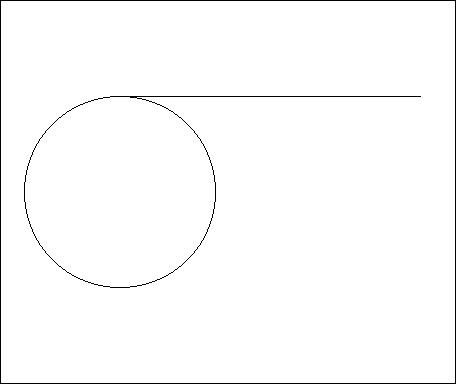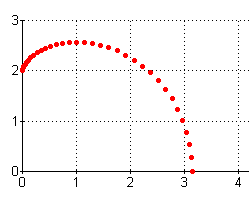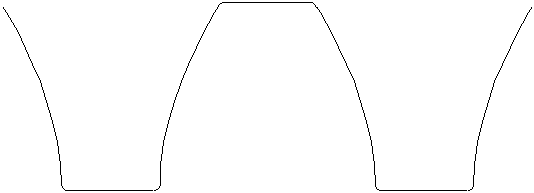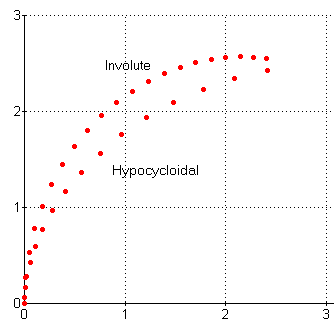## The Involute Curve

There is another approach used to simulate rolling action. Consider what happens when you place one end of a straight line (with a length equal to half the circumference of the circle) on the perimeter of a circle and then rotate that line around the perimeter of the circle until the other end touches the perimeter of the circle.If you observe what happens to the end of the line, you will see how it moves away from the circle. If you trace a line along the path taken by the end of the straight line as it rolls around the circle, the result is an Involute Curve (with Displacement in Inches on the both axes).Select this link to see the chart that was used to create this graph.

The main difference in this design is that the entire tooth is based upon the involute curve rather than just the addendum. The shape of the involute curve is determined by the size (radius) of the generating circle (that the straight line rolls around). Since the entire tooth is based upon the involute curve, the tooth flank (the lower part, below the pitch circle) does not point to the circle center (as happens in the radial flanks): the tooth is wider at the base and is therefore much stronger by design.The portion of the Involute Curve that would be used to design a clock gear tooth is steeper (it has a larger gradient) than the Epicycloidal Curve. A portion of the two curves is compared in the graph below. The axes both indicate displacement in inches. Since the radius of the generating circle is one, the X axis also indicates the angle in radians for the Epicycloidal Curve. The portion of the curves used to design a clock gear tooth would be equivalent to a rotation of 60ş (if a 6 tooth pinion were used) or p/3 (=1.05) radians. To compare the curves, I used a mirror image of the Involute Curve by subtracting the X values from p before creating the graph (with Displacement in Inches on the both axes).Note that one way to strengthen the tooth design with the epicycloidal design would be to design the flank based upon a hypocycloidal curve, which is defined as a curve that is generated by rolling a circle around the circumference inside another circle (compare with the epicycloidal curve, rolling a circle around the circumference outside another circle). The result is that the tooth space (between two gear teeth) has a somewhat more similar shape below the pitch circle (in the dedendum portion) to the shape of the addendum of the tooth of the other gear that goes into it. Go to Module and Gear Cutters Clock Repair Main Page Escapements in Motion Links Page